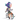# 常用排序算法之JavaScript实现

January 16, 2016

### 冒泡排序

##### 算法描述和实现

• 比较相邻的元素。如果第一个比第二个大，就交换它们两个；
• 对每一对相邻元素作同样的工作，从开始第一对到结尾的最后一对，这样在最后的元素应该会是最大的数；
• 针对所有的元素重复以上的步骤，除了最后一个；
• 重复步骤1~3，直到排序完成。
##### JavaScript代码实现：
``````Array.prototype.bubble_sort = function() {
var i, j, temp;
for (i = 0; i < this.length - 1; i++)
for (j = 0; j < this.length - 1 - i; j++)
if (this[j] > this[j + 1]) {
temp = this[j];
this[j] = this[j + 1];
this[j + 1] = temp;
}
return this;
};``````

### 选择排序

##### 算法描述和实现

n个记录的直接选择排序可经过n-1趟直接选择排序得到有序结果。具体算法描述如下：

• 初始状态：无序区为R[1..n]，有序区为空；
• 第i趟排序(i=1,2,3…n-1)开始时，当前有序区和无序区分别为R[1..i-1]和R(i..n）。该趟排序从当前无序区中选出关键字最小的记录 R[k]，将它与无序区的第1个记录R交换，使R[1..i]和R[i+1..n)分别变为记录个数增加1个的新有序区和记录个数减少1个的新无序区；
• n-1趟结束，数组有序化了。
##### JavaScript代码实现：
``````Array.prototype.selection_sort = function() {
var i, j, min;
var temp;
for (i = 0; i < this.length - 1; i++) {
min = i;
for (j = i + 1; j < this.length; j++){
if (this[min] > this[j]){
min = j;
}
}
temp = this[min];
this[min] = this[i];
this[i] = temp;
}
return this;
};``````

### 插入排序

##### 算法描述和实现

• 从第一个元素开始，该元素可以认为已经被排序；
• 取出下一个元素，在已经排序的元素序列中从后向前扫描；
• 如果该元素（已排序）大于新元素，将该元素移到下一位置；
• 重复步骤3，直到找到已排序的元素小于或者等于新元素的位置；
• 将新元素插入到该位置后；
• 重复步骤2~5。
##### JavaScript代码实现：
``````Array.prototype.insertion_sort = function() {
var i, j;
var temp;
for (i = 1; i < this.length; i++) {
temp = this[i];
for (j = i - 1; j >= 0 && this[j] > temp; j--){
this[j + 1] = this[j];
}
this[j + 1] = temp;
}
return this;
};``````

### 归并排序

##### 算法描述和实现

• 把长度为n的输入序列分成两个长度为n/2的子序列；
• 对这两个子序列分别采用归并排序；
• 将两个排序好的子序列合并成一个最终的排序序列。
##### JavaScript代码实现：
``````Array.prototype.merge_sort = function() {
var merge = function(left, right) {
var final = [];
while (left.length && right.length)
final.push(left <= right ? left.shift() : right.shift());
return final.concat(left.concat(right));
};
var len = this.length;
if (len < 2) return this;
var mid = len / 2;
return merge(this.slice(0, parseInt(mid)).merge_sort(), this.slice(parseInt(mid)).merge_sort());
};``````

### 快速排序

##### 算法描述和实现

• 从数列中挑出一个元素，称为 “基准”（pivot）；
• 重新排序数列，所有元素比基准值小的摆放在基准前面，所有元素比基准值大的摆在基准的后面（相同的数可以到任一边）。在这个分区退出之后，该基准就处于数列的中间位置。这个称为分区（partition）操作；
• 递归地（recursive）把小于基准值元素的子数列和大于基准值元素的子数列排序。
##### JavaScript代码实现：
``````Array.prototype.quick_sort = function() {
var len = this.length;
if (len <= 1)
return this.slice(0);
var left = [];
var right = [];
var mid = [this];
for (var i = 1; i < len; i++)
if (this[i] < mid)
left.push(this[i]);
else
right.push(this[i]);
return left.quick_sort().concat(mid.concat(right.quick_sort()));
};``````
##### 算法分析